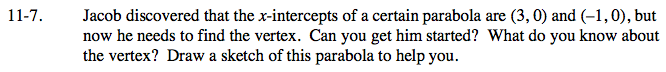Home > AC > Chapter 11 > Lesson 11.1.1 > Problem11-7

11-7.

Jacob discovered that the x-intercepts of a certain parabola are (3, 0) and (−1, 0), but now he needs to find the vertex. Can you get him started? What do you know about the vertex? Draw a sketch of this parabola to help you. Homework Help ✎Sketch the parabola on your graph paper first.

Remember that parabolas are symmetric shapes and the vertex is on the line of symmetry.
Knowing this information, what part of the vertex do you know?